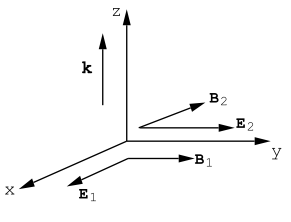$$\require{cancel}$$

# 9.2: Phasors

It is very convenient to represent sinusoidal functions i.e. sines and cosines, by complex exponential functions when dealing with linear differential equations such as Maxwell’s equations. For example

$\text{y}=\text{A} \exp [i(\text{kx}-\omega \text{t})]\nonumber$

means

$\text{y}=\text {Real Part}(\text{A} \exp [i(\text{kx}-\omega \text{t})])=\text{A} \cos (\text{kx}-\omega \text{t})\nonumber$

if A is a real number, or if A= a+ib is a complex number

\begin{align} \text{y} &=\operatorname{Real} \operatorname{Part}((\text{a}+\text{ib}) \exp [i(\text{kx}-\omega \text{t})]), \label{9.16} \\ &=\text{a} \cos (\text{kx}-\omega \text{t})-\text{b} \sin (\text{kx}-\omega \text{t}).\nonumber \end{align}

A complex amplitude represents a phase shift. Since

$\cos (\alpha+\beta)=\cos \beta \cos \alpha-\sin \beta \sin \alpha, \label{9.17}$

Equation (\ref{9.16}) can be written

$y=\sqrt{a^{2}+b^{2}} \cos (k x-\omega t+\beta),\nonumber$

where

$\sin \beta=\frac{b}{\sqrt{a^{2}+b^{2}}}\nonumber$

and

$\cos \beta=\frac{\text{a}}{\sqrt{\text{a}^{2}+\text{b}^{2}}},$

or

$\tan \beta=\frac{b}{a}.$

In phasor notation

$\text{y}=\sqrt{\text{a}^{2}+\text{b}^{2}} \exp [i(\text{kx}-\omega \text{t}+\beta)]=(\sqrt{\text{a}^{2}+\text{b}^{2}} \exp i \beta) \exp [i(\text{kx}-\omega \text{t})].\nonumber$

The prefactor $$(\sqrt{a^{2}+b^{2}} \exp i \beta)$$ is just the polar representation of the complex number (a+ib).

Derivatives are particularly convenient in the complex phasor notation because the derivative of an exponential function gives back the same exponential function multiplied by a constant (usually a complex number).

One must be careful when calculating energy densities or when calculating the Poynting vector using the phasor notation because the Real Part of the product of two complex exponentials is not the same as the product of the two Real sinusoidal functions that appear in the product. There is, however, a trick which is useful. Consider a plane wave propagating along z and which can be described by

$\text{E}_{\text{x}}=\text{E}_{0} \text{e}^{i\left(\text{kz}-\omega t+\phi_{1}\right)} \label{9.18}$

$\text{H}_{y}=\text{H}_{0} \text{e}^{i\left(\text{k} z-\omega t+\phi_{2}\right)} \nonumber$

These electric and magnetic fields are not in phase because $$\phi_{1}$$ and $$\phi_{2}$$ are different, and therefore this plane wave is not propagating in free space. It corresponds to a wave propagating in a medium characterized by a complex dielectric constant as will be discussed in a later chapter. Now calculate the time average of the Poynting vector, $$\vec{\text{S}}=\vec{\text{E}} \times \vec{\text{H}}$$, using Equations (\ref{9.18}). It is asserted that the time average of the product of two phasors can be obtained as one-half of the real part of the product of one phasor with the complex conjugate of the other phasor.

Thus

$<\text{S}_{z}>=\frac{1}{2} \operatorname{Real}\left(\text{E}_{\text{x}} \text{H}_{y}^{*}\right)=\frac{1}{2} \operatorname{Real}\left(\text{E}_{\text{x}}^{*} \text{H}_{y}\right), \label{9.19}$

where $$E_{x}^{*}$$ means the complex conjugate of Ex, and $$\text{H}_{\text{y}}^{*}$$ means the complex conjugate of Hy. Using Equation (\ref{9.18}) in Equation (\ref{9.19}) one obtains

$<\text{S}_{z}>=\frac{1}{2} \operatorname{Real}\left(\text{E}_{0} \text{H}_{0} \exp i\left(\phi_{1}-\phi_{2}\right)\right)=\frac{\text{E}_{0} \text{H}_{0}}{2} \cos \left(\phi_{1}-\phi_{2}\right), \label{9.20}$

since E0, H0 are taken to be real amplitudes. Eqn.(\ref{9.19}) can be checked by writing the fields (\ref{9.18}) in real form:

$\text{S}_{z}=\text{E}_{0} \text{H}_{0} \cos \left(\text{kz}-\omega \text{t}+\phi_{1}\right) \cos \left(\text{kz}-\omega \text{t}+\phi_{2}\right), \nonumber$

or, using Equation (\ref{9.17}),

\begin{aligned} \text{S}_{\text{z}}=& \text{E}_{0} \text{H}_{0}\left(\cos \phi_{1} \cos (\text{kz}-\omega \text{t})-\sin \phi_{1} \sin (\text{kz}-\omega \text{t})\right) \\ & \times\left(\cos \phi_{2} \cos (\text{kz}-\omega \text{t})-\sin \phi_{2} \sin (\text{kz}-\omega \text{t})\right), \end{aligned}

or upon an explicit multiplication

\begin{aligned} \text{S}_{\text{z}}=& \text{E}_{0} \text{H}_{0}\left(\cos \phi_{1} \cos \phi_{2} \cos ^{2}(\text{kz}-\omega \text{t})-\cos \phi_{2} \sin \phi_{1} \sin (\text{kz}-\omega \text{t}) \cos (\text{kz}-\omega \text{t})\right) \\ &-\text{E}_{0} \text{H}_{0}\left(\sin \phi_{2} \cos \phi_{1} \sin (\text{kz}-\omega \text{t}) \cos (\text{kz}-\omega \text{t})-\sin \phi_{1} \sin \phi_{2} \sin ^{2}(\text{kz}-\omega \text{t})\right) \end{aligned}

Upon taking the time averages one obtains

$<\text{S}_{z}>=\frac{\text{E}_{0} \text{H}_{0}}{2}\left(\cos \phi_{1} \cos \phi_{2}+\sin \phi_{1} \sin \phi_{2}\right).\nonumber$

This equation can be written compactly as

$<\text{S}_{\text{z}}>=\frac{\text{E}_{0} \text{H}_{0}}{2} \cos \left(\phi_{1}-\phi_{2}\right), \nonumber$

in agreement with the result Equation (\ref{9.20}) obtained using the prescription (\ref{9.19}).Figure $$\PageIndex{3}$$: Two coherent plane waves having orthogonal polarizations, and propagating along the z-direction. Each wave is characterized by the same circular frequency, ω, and the same wave-vector, $$\vec k$$, where $$\text{k}_{\text{z}}=|\vec{\text{k}}|=\omega / \text{c}$$. Let the fields in wave number (2) be shifted in phase by $$\phi$$ radians relative to the fields in wave number (1).